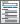Home  /  Products  /  Features  /  Linear models

## Linear models

Linear regressionWatch Fitting and interpreting regression models tutorials

Censored outcomes

Sample-selection linear models• Linear and exponential
• Lower or upper boundary values
• Robust, cluster—robust, bootstrap, and jackknife standard errors

Stochastic frontier models• Production and cost frontiers
• Half-normal, exponential, and truncated-normal distributions
• Modeling of conditional heteroskedasticity
• Median regression
• Regression of any quantile
• Interquantile range regression
• Standard errors
• Koenker and Bassett
• Robust — choose bandwidth and kernel
• Bootstrap
• Multiple imputation
• Support for a wide variety of models
• Component-plus-residual plots
• Support for zero-inflated regressors

Endogeneity and simultaneous systems

• Inverse quantile regression estimator
• Smoothed estimating equations estimator
• Simultaneously estimate at different quantiles
• Hypothesis testing of endogenous effects
• Confidence intervals robust to weak instruments

Seemingly unrelated regressions• Robust (Huber/White/sandwich) and cluster–robust standard errors New
• Linear constraints within and across equations
• View and run all postestimation features for your command
• Automatically updated as estimation commands are run
• Automatically create indicators based on categorical variables
• Form interactions among discrete and continuous variables
• Include polynomial terms
• Perform contrasts of categories/levels
Watch Introduction to factor variables in Stata tutorials
Watch Introduction to margins in Stata tutorials
Watch Profile plots and interaction plots in Stata tutorials
• Analysis of main effects, simple effects, interaction effects, partial
• interaction effects, and nested effects
• Comparisons against reference groups, of adjacent levels, or against
• the grand mean
• Orthogonal polynomials
• Helmert contrasts
• Custom contrasts
• ANOVA-style tests
• Contrasts of nonlinear responses
• Balanced and unbalanced data
• Contrasts in odds-ratio metric
• Contrasts of means, intercepts, and slopes
• Graphs of contrasts• Compare estimated means, intercepts, and slopes
• Compare marginal means, intercepts, and slopes
• Balanced and unbalanced data
• Nonlinear responses
• Multiple-comparison adjustments: Bonferroni, Šidák, Scheffé, Tukey HSD, Duncan, and Student–Newman–Keuls adjustments
• Group comparisons that are significant
• Graphs of pairwise comparisons